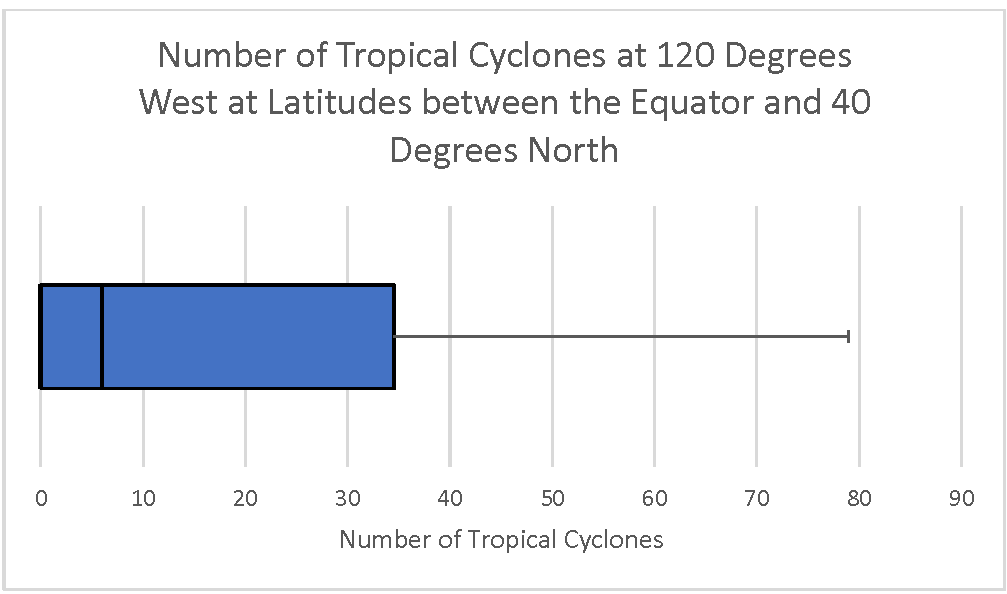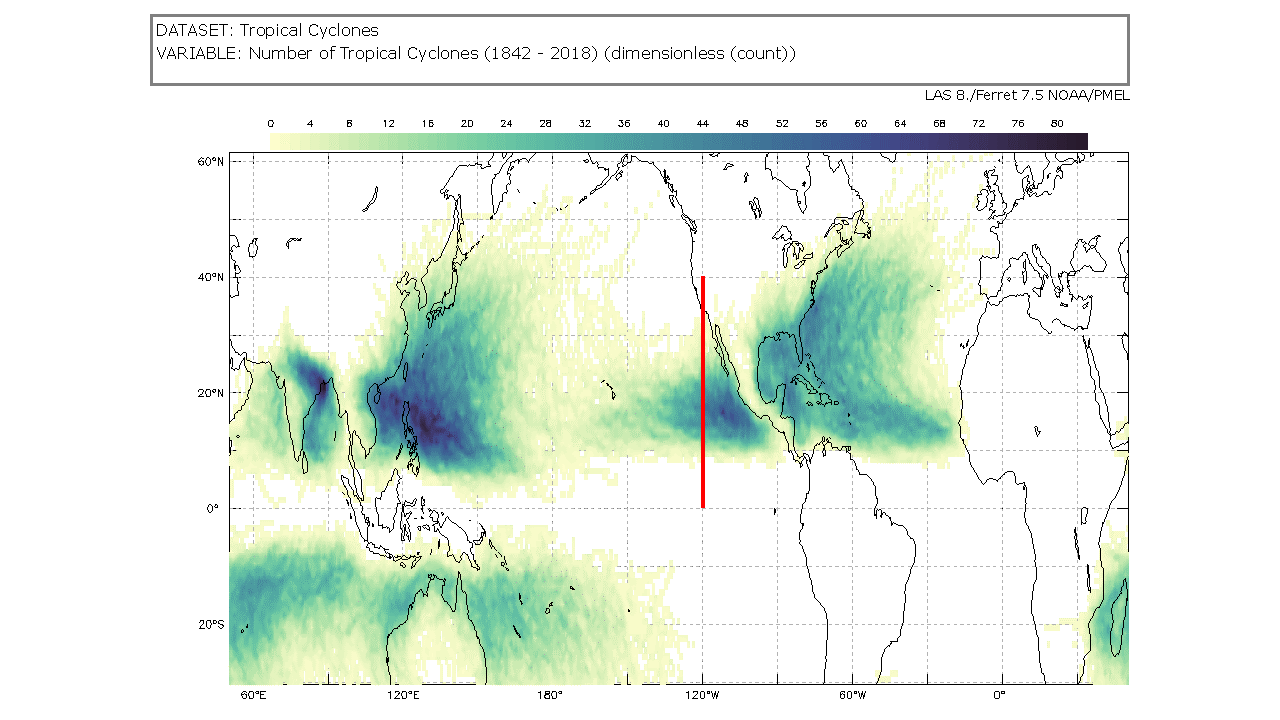# Tropical Cyclone Counts Box Plot

### Overview

Learners will analyze and interpret a box plot and evaluate the spread of the data. Learners will compare it with a different visualization of the data to see how the two compare, discuss the limitations of the two types of data displays and formulate questions.

### Student Directions

Tropical cyclones are sometimes called hurricanes or typhoons. These data may be visualized in a variety of ways.

Below you will find two types of visualizations of the same data, a box plot and a map. You will analyze these and extract information. Recall, a box plot is a type of graph A box plot is a type of graph which shows variability within a group. It shows the minimum, maximum, median, range, upper and lower quartile of the data. One may use a box plot to answer specific kinds of questions about their data.

• What is the range of the data?
• What is the median of the data?
• What is the range for the top 25% of the data? The bottom 25%?
• What is the maximum/minimum of the data?

#### Steps

1. The box plot below shows the number of tropical cyclones at 120 degrees west for each degree of latitude from the equator to 40 degrees north; the same as represented along the red line in the mapped image below.
2. Analyze the box plot and answer the questions that follow. Check with your instructor on how to submit answers.Number of Cyclones at 120 degrees west at latitudes between the equator and 40 degrees north https://mynasadata.larc.nasa.gov/sites/default/files/inline-images/Box%20Plot%20120%20West%20horizontal.png
1. What does the box plot show? What does it NOT show?
2. What is the overall distribution of the box plot? Is it skewed right (with a longer whisker on the right)? Skewed left (with a longer whisker on the left)?
3. What does the shape of the distribution tell you?
4. What are the maximum, minimum, range, median, first quartile, third quartile, and interquartile range?
3. The map below shows is a thick line at 120 degrees west from the equator to 40 degrees north; the same locations represented in the box plot. (The Tropical Cyclone Counts map was generated in the My NASA Data Earth System Data Explorer. ) Now, compare the box plot to the map image and answer the following questions.Tropical Cyclone Counts Map showing a line at 120 degrees west from the equator to 40 degrees north https://mynasadata.larc.nasa.gov/sites/default/files/2022-02/Tropical%20Cyclone%20count%20120%20W.png
1. Which visualization shows the number of tropical cyclones at each latitude?
3. What do you wonder from the box plot? Can you answer it with the box plot, or do you need to see the data in a different way?

### Teacher Note

Teachers, these mini lessons/student activities are perfect "warm up" tasks that can be used as a hook, bell ringer, exit slip, etc. They take less than a class period to complete. Learn more on the "My NASA Data What are Mini Lessons?" page.

Teachers who are interested in receiving the answer key, please complete the Teacher Key Request and Verification Form. We verify that requestors are teachers prior to sending access to the answer keys as we’ve had many students try to pass as teachers to gain access.

• 6-8

• 30 minutes